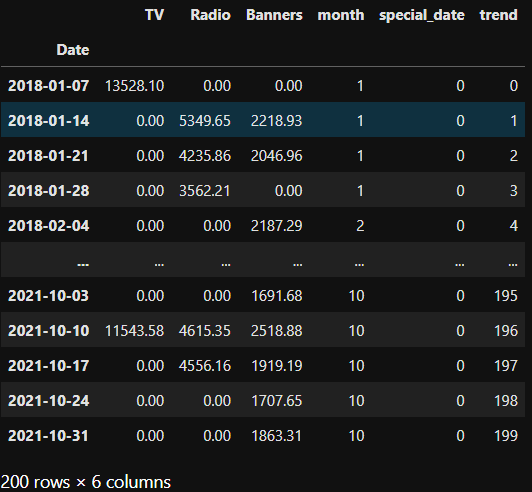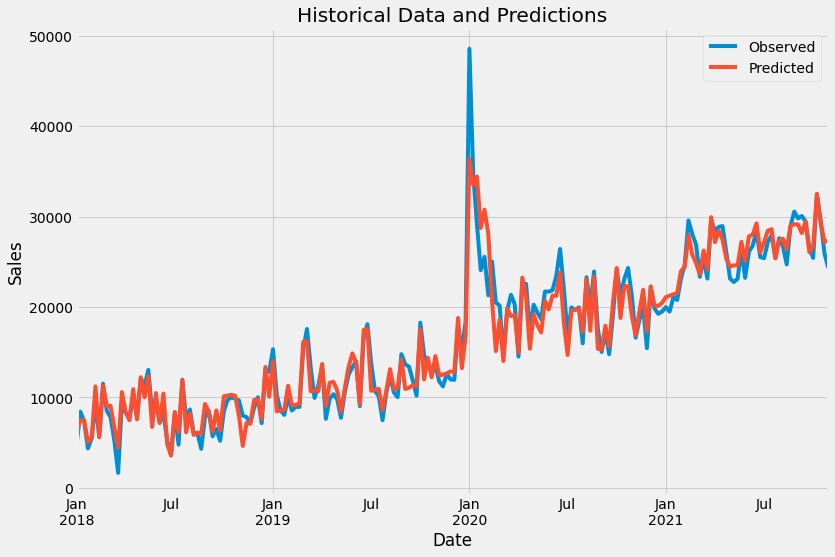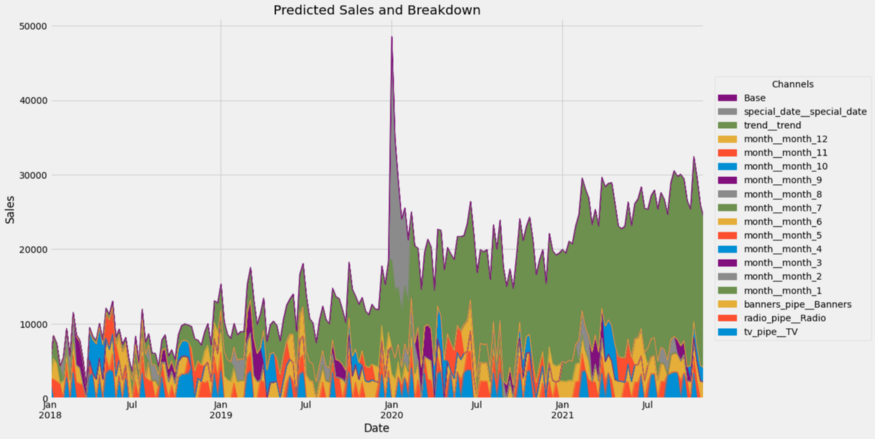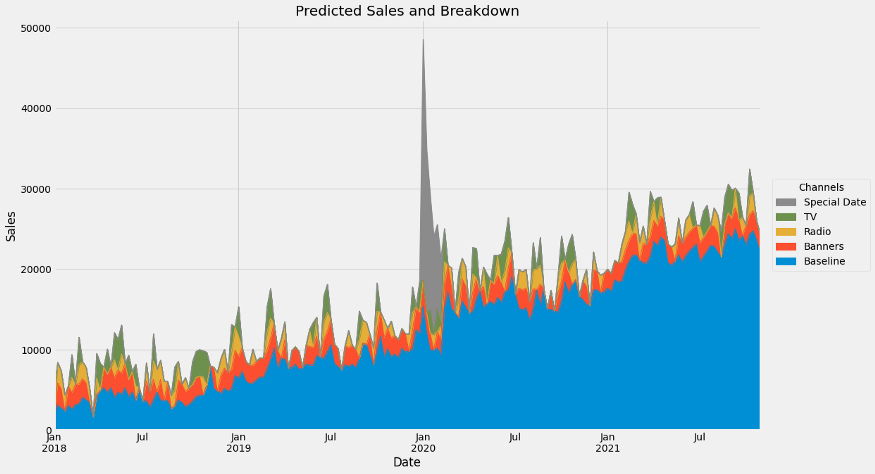# MaMiMo

This is a small library that helps you with your everyday Marketing Mix Modelling. It contains a few saturation functions, carryovers and some utilities for creating with time features. You can also read my article about it here: >>>Click<<<.

Give it a try via `pip install mamimo`!

# Small Example

You can create a marketing mix model using different components from MaMiMo as well as scikit-learn. First, we can create a dataset via

```from mamimo.datasets import load_fake_mmm

X = data.drop(columns=['Sales'])
y = data['Sales']```

`X` contains media spends only now, but you can enrich it with more information.

## Feature Engineering

MaMiMo lets you add time features, for example, via

```from mamimo.time_utils import add_time_features, add_date_indicators

X = (X
.assign(trend=range(200))
)```

• a month column (integers between 1 and 12),
• a binary column named special_date that is 1 on the 5h of January 2020 and 0 everywhere else, and
• a (so far linear) trend which is only counting up from 0 to 199.

`X` looks like this now:## Building a Model

We can now build a final model like this:

```from mamimo.time_utils import PowerTrend
from sklearn.preprocessing import OneHotEncoder

cats =  [list(range(1, 13))] # different months, known beforehand

preprocess = ColumnTransformer(
[
('tv_pipe', Pipeline([
('carryover', ExponentialCarryover()),
('saturation', ExponentialSaturation())
]), ['TV']),
('carryover', ExponentialCarryover()),
('saturation', ExponentialSaturation())
('banners_pipe', Pipeline([
('carryover', ExponentialCarryover()),
('saturation', ExponentialSaturation())
]), ['Banners']),
('month', OneHotEncoder(sparse=False, categories=cats), ['month']),
('trend', PowerTrend(), ['trend']),
('special_date', ExponentialCarryover(), ['special_date'])
]
)

model = Pipeline([
('preprocess', preprocess),
('regression', LinearRegression(
positive=True,
fit_intercept=False # no intercept because of the months
)
)
])```

This builds a model that does the following:

• the media channels are preprocessed using the adstock transformation, i.e. a carryover effect and a saturation is added
• the month is one-hot (dummy) encoded
• the trend is changed from linear to something like t^a, with some exponent a to be optimized
• the special_date 2020-01-05 gets a carryover effect as well, meaning that not only on this special week there was some special effect on the sales, but also the weeks after it

## Training The Model

We can then hyperparameter tune the model via

```from sklearn.model_selection import RandomizedSearchCV, TimeSeriesSplit

tuned_model = RandomizedSearchCV(
model,
param_distributions={
'preprocess__tv_pipe__carryover__window': randint(1, 10),
'preprocess__tv_pipe__carryover__strength': uniform(0, 1),
'preprocess__tv_pipe__saturation__exponent': uniform(0, 1),
'preprocess__banners_pipe__carryover__window': randint(1, 10),
'preprocess__banners_pipe__carryover__strength': uniform(0, 1),
'preprocess__banners_pipe__saturation__exponent': uniform(0, 1),
'preprocess__trend__power': uniform(0, 2),
'preprocess__special_date__window': randint(1, 10),
'preprocess__special_date__strength': uniform(0, 1),
},
cv=TimeSeriesSplit(),
random_state=0,
n_iter=1000, # can take some time, lower number for faster results
)```

You can also use `GridSearch`, Optuna, or other hyperparameter tune methods and packages here, as long as it is compatible to scikit-learn.

## Analyzing

With `tuned_model.predict(X)` and some plotting, we getYou can get the best found hyperparameters using `print(tuned_model.best_params_)`.

### Plotting

You can compute the channel contributions via

```from mamimo.analysis import breakdown

contributions = breakdown(tuned_model.best_estimator_, X, y)```

This returns a dataframe with the contributions of each channel fo each time step, summing to the historical values present in `y`. You can get a nice plot via

```ax = contributions.plot.area(
figsize=(16, 10),
linewidth=1,
title="Predicted Sales and Breakdown",
ylabel="Sales",
xlabel="Date",
)
handles, labels = ax.get_legend_handles_labels()
ax.legend(
handles[::-1],
labels[::-1],
title="Channels",
loc="center left",
bbox_to_anchor=(1.01, 0.5),
)```Wow, that’s a lot of channels. Let us group some of them together.

```group_channels = {'Baseline': [f'month__month_{i}' for i in range(1, 13)] + ['Base', 'trend__trend']}
# read: 'Baseline consists of the months, base and trend.'
# You can add more groups!

contributions = breakdown(
tuned_model.best_estimator_,
X,
y,
group_channels
)```

If we plot again, we getYay!

View Github